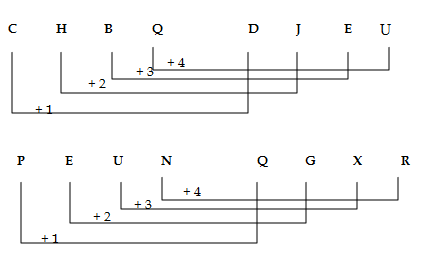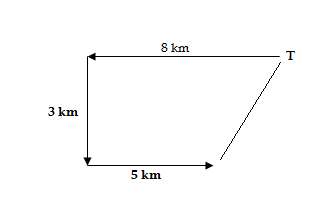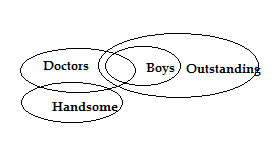# SSC CGL 2018 Practice Test Papers | Reasoning Ability (Day-60)

Dear Aspirants, Here we have given the Important SSC CGL Exam 2018 Practice Test Papers. Candidates those who are preparing for SSC CGL 2018 can practice these questions to get more confidence to Crack SSC CGL 2018 Examination.

[WpProQuiz 3753]

Click “Start Quiz” to attend these Questions and view Explanation

1) In the following question, select the related word pair from the given alternatives.

CHBQ : DJET : : PEUN : ?

a) TUVW

b) PQRS

c) QGXR

d) GQXR

2) In the following question, select the related number from the given alternatives.

9 :729 : : 5 ?

a) 250

b) 125

c) 121

d) 25

3) In the following question, select the odd word from the given alternatives.

a) Eyes

b) Legs

c) Nose

d) Hands

4) In the following question, select the odd number pair from the given alternatives.

a) 23 – 92

b) 14 – 71

c) 15 – 120

d) 17 – 51

5) Arrange the given words in the sequence in which they occur in the dictionary.

i) Spring

ii) Slim

iii) Smooth

iv) Summer

a) ii, iii, i, iv

b) ii, i, iv, iii

c) ii, iii, iv, i

d) i, iii, iv, ii

6) In the following question, select the missing number from the given series.

1, 1, 4, 3, 9, 5, 16, ?, 25,  9, 36, 11, 49,  13

a) 15

b) 7

c) 8

d) 4

7) Sanjay started from point T and walked straight 8 km west, then turned left and walked 3km and again turned left and walked straight 5 km. In which direction is he from T?

a) North East

b) South West

c) North West

d) South East

8) If 28 # 59 = 24 and 86 # 61 = 21, then 77 # 88 =?

a) 45

b) 32

c) 30

d) 42

9) How many triangles are there in given figure?

a) 12

b) 11

c) 9

d) 10

10) In each of the following question below are given some statements followed by some conclusions. Taking the given statements to be true even if theyseem to be at variance from commonly known facts, read all the conclusions and then decide which of the given conclusion logically follows the given statements.

Statements:

1. All boys are Outstanding Students
2. Some Doctors are Boys
3. Some Doctors are handsome

Conclusion

1. All Doctors are handsome
2. All outstanding students are Doctors
3. Some Doctors are outstanding students

a) Only I follow

b) Only III follow

c) Only I and III follow

d) All follow93 = 729

53 = 125

Except Nose others are parts are pairs.

Second number is a multiple of first number

ii) Slim

iii) Smooth

i) Spring

iv) Summer

1, 1, 4, 3, 9, 5, 16, 7, 25, 9, 36, 11, 49, 1328 # 59 = 24

(2 + 8) + (5+ 9) = 10 + 14 = 24

86 # 61

(8+ 6) + (6 + 1) = 14 + 7 = 21

77 # 88

(7 + 7) + (8 + 8) = 14 + 16 = 30